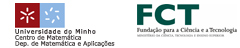EN || PT || ESAna Romero (Universidad de la Rioja)Effective homology of groups and discrete Morse theory

In the talk we will present several algorithms related with the computation of the homology of groups, by means of different techniques of Algebraic Topology. More concretely, we have developed some algorithms which, making use of the effective homology method, construct the homology groups of Eilenberg-MacLane spaces K(G,1) for different groups G, allowing one in particular to determine the homology groups of G. These results can be applied to the computation of homology groups of central extensions and 2-types. Moreover, our initial algorithms have been improved by using discrete Morse theory (concretely, by constructing discrete vector fields which describe the homology of finite cyclic groups).© 3º Encontro Ibérico de Matemática :: 2010